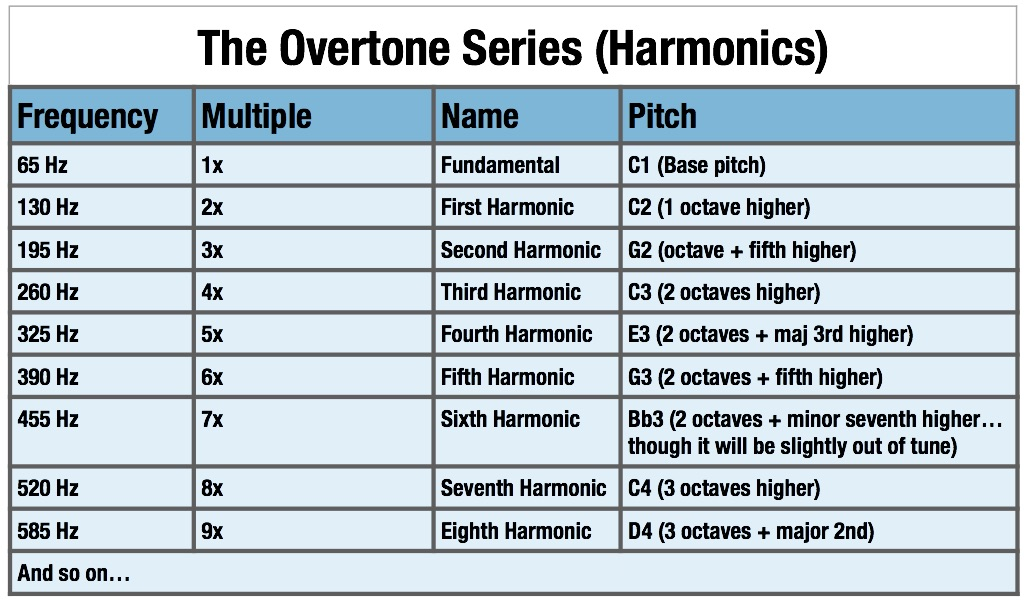# Vibrating Rod Harmonics

John Tyndall
Rod Fixed at Both Ends
"A rod fixed at both ends and caused to vibrate transversely divides itself in the same manner as a string vibrating transversely.

"But the succession of its overtones is not the same as those of a string, for while the series of tones emitted by the string is expressed by the natural numbers, 1, 2, 3, 4, 5, etc., the series of tones emitted by the rod is expressed by the squares of the odd numbers, 3, 5, 7, 9, etc." [from "Sound" by John Tyndall]

Rod Fixed at One End
"A rod fixed at one end can also vibrate as a whole, or can divide itself into vibrating segments separated from each other by nodes.

"In this case the rate of vibration of the fundamental tone is to that of the first overtone as 4:25, or as the square of 2 to the square of 5. From the first division onwards the rates of vibration are proportional to the squares of the odd numbers, 3, 5, 7, 9, etc.

"With rods of different lengths the rate of vibration is inversely proportional to the square of the length of the rod." [from "Sound", John Tyndall]

Ramsay
pendulum where fourth the length is double the oscillations. A third condition in this order is in springs or reeds where half the length is four times the vibrations. If we take a piece of straight wire and make it oscillate as a pendulum, one-fourth will give double the oscillations; if we fix it at one end, and make it vibrate as a spring, half the length will give four times the vibrations; if we fix it at both ends, and make it vibrate as a musical string, half the length will produce double the number of vibrations per second. [Scientific Basis and Build of Music, page 80]Harmonic Series